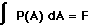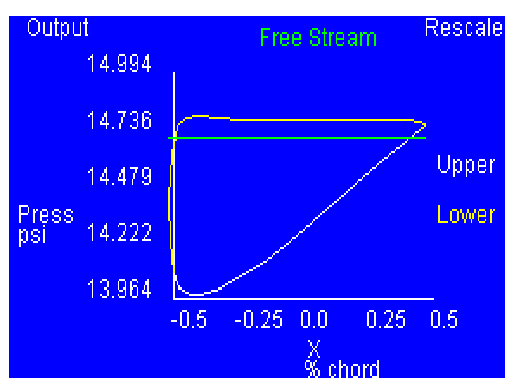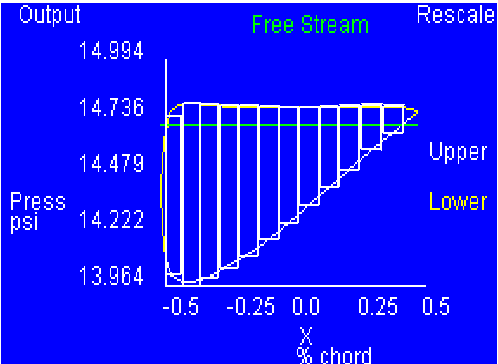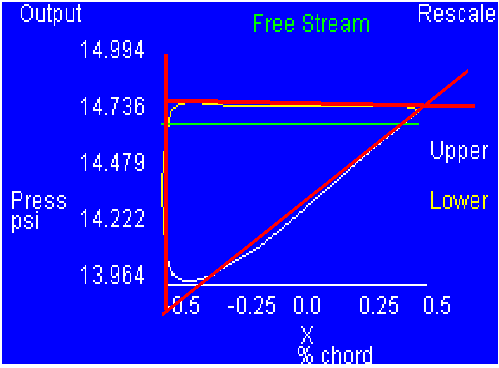+ Text Only Site
+ Non-Flash Version
+ Contact Glenn## Getting a Little "Lift" out of Calculus Part I: ActivityIf so instructed by your teacher, print out a worksheet page for these problems.

 Background: Pressure is the change in force per unit of area and can be represented as the derivative P(A) = (d/dA)F or P(A) = dF/dA Therefore, force is the integral of the pressure with respect to area, written asTo find the net lift force provided by an airfoil with a given set of parameters, subtract the force pushing down on the upper surface from the force pushing up on the lower surface. This causes the integral to becomewhere PL(A) represents the pressure function acting on the lower surface and PU(A) represents the pressure function acting on the upper surface. Procedure: Using FoilSim, set the following conditions: Airspeed = 75 mph Altitude = 0 ft Angle = 20 degrees Thickness = 25 % Camber = 17.5 % Chord = 1 ft Span = 1 ft Use command-shift-3 (on a Macintosh) or use the "Alt-Print Screen" or "prtscr" button (on Windows) to screen-capture the page. Paste the graph in a word processing or paint document. Crop the picture so that just the graph remains as shown in Figure 1. Use the printing options to enlarge and print the graph.Figure 1 Be careful with scaling. Since the Chord setting in the FoilSim control panel was set at 1 ft., the curves displayed will be from 0 inches to 12 inches, and the area along the X-axis goes from 0 to 144 sq in. Draw narrow rectangles with equal widths across the region bounded by the curves (as shown in Figure 2) and find the area of each. (Be careful with the scaling.) Create a table and record this information.Figure 2 The total area between the curves is the sum of the individual areas; this value represents the lift force. Compare your value to the value given by FoilSim. Using the same graph, draw a straight line along the curves as shown in Figure 3.Figure 3 Find the slopes of each line and use them to find the respective equations. Use integration techniques to find the area of the enclosed region; this value represents the lift force. Compare your value to the value given by FoilSim and to your previous value.

Related Pages:
Standards
Worksheet
Lesson Index
Aerodynamics Index+ Inspector General Hotline + Equal Employment Opportunity Data Posted Pursuant to the No Fear Act + Budgets, Strategic Plans and Accountability Reports + Freedom of Information Act + The President's Management Agenda + NASA Privacy Statement, Disclaimer, and Accessibility CertificationEditor: Tom Benson NASA Official: Tom Benson Last Updated: May 13 2021 + Contact Glenn